# Detectors and descriptors of the FAST, BRIEF, ORB

In this article, we will discuss some algorithms for searching and describing singular points of images. Here this topic is already rose , and more than once . I will assume that the basic definitions of the reader are already familiar, we will examine in detail the heuristic algorithms FAST, FAST-? FAST-ER, BRIEF, rBRIEF, ORB, and discuss the sparkling ideas underlying them. In part, this will be a free translation of the essence of several articles[1,2,3,4,5], there will be little code to "try."here

Variable parameters (described in the code): radius of the circle (takes values ​​??3), parameter n (in the original n = 12), parameter t. The code opens the file in.bmp, processes the image, saves it in out.bmp. Pictures usual 24-bit.

# We are building a decision tree, Tree FAST, FAST-9

In 200? the workmanaged to develop the original idea using machine learning and decision trees.

The original FAST has the following drawbacks:

Several adjacent pixels can be marked as special points. We need some measure of the "strength" of the feature. One of the first measures proposed is the maximum value of t, at which the point is still taken as a special point.

A quick 4-point test does not generalize for n less than 12. For example, visually the best results of the method are achieved at n = ? not 12.

I would like to speed up the algorithm!

Instead of using a cascade of two tests from 4 and 16 points, it is suggested to do everything in one go through the decision tree. Similar to the original method, we compare the brightness of the central point with the points on the circle, but in this order, to make a decision as quickly as possible. And it turns out that you can make a decision in just ~ 2 (!!!) comparisons on average.

The very best is how to find the order you need to compare points. Find with machine learning. Suppose someone has noted for us on the picture a lot of special points. We will use them as a set of training examples and the idea is that as the next point greedy choose the one that will give the most information at this step. For example, let initially in our sample there were 5 singular points and 5 NON-singular points. In the form of a tablet like this:We now choose one of the pixels p of the circle and for all singular points we compare the central pixel with the selected one. Depending on the brightness of the selected pixel near each singular point, the table can have the following result: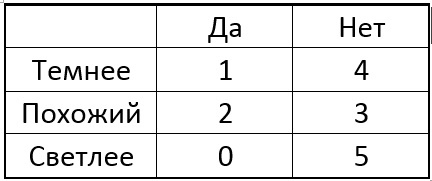The idea is to select a point p so that the numbers in the columns of the table differ as much as possible. And if now for the new unknown point we get the result of the comparison "Lighter", then we can immediately say that the point is "not special" (see the table). The process continues recursively until points of only one of the classes fall into each group after the division into "darker-similar-lighter". The result is a tree of the following form: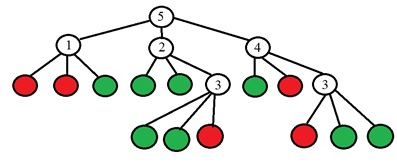In the leaves of the tree there is a binary value (red - special, green - not special), and in the remaining vertices of the tree there is a number of the point that needs to be analyzed. More specifically, in the original article, it is proposed to make a choice of the point number from the entropy change. The entropy of the set of points is calculated:

\$\$ display \$\$ H = left ({c + overline c} right) {log _2} left ({c + overline c} right) - c {log _2} c - overline c {log _2} overline c \$\$ display \$\$

c is the number of singular points,
\$ inline \$ {bar c} \$ inline \$
Is the number of non-singular points of the set

Change in entropy after processing of the point p:

\$\$ display \$\$ Delta H = H - {H_ {dark}} - {H_ {equal}} - {H_ {bright}} \$\$ display \$\$

Accordingly, a point is chosen for which the entropy change will be maximum. The process of partitioning stops when the entropy is zero, which means that all points are either singular or vice versa - all are not special. With software implementation, after all this, the decision tree is transformed into a set of "if-else" constructs.

The last step in the algorithm is the suppression of the non-maxima, in order to obtain one of several nearby points. The developers propose to use the original measure based on the sum of the absolute differences between the center point and the points of the circle in this form:

\$\$ display \$\$ V = max left {{sumlimits_ {x in {S_ {bright}}} {left | {{I_x} - {I_p}} right | - t, sumlimits_ {x in {S_ {dark}}} {left | {{I_p} - {I_x}} right | - t}}} right) \$\$ display \$\$

Here
\$ inline \$ {S_ {bright}} \$ inline \$
and
\$ inline \$ {S_ {dark}} \$ inline \$
respectively, the group of pixels is lighter and darker, t is the threshold value of brightness,
\$ inline \$ {I_p} \$ inline \$
- brightness of the central pixel,
\$ inline \$ {{I_x}} \$ inline \$
- the brightness of the pixel on the circle. You can try the algorithm with the following code . The code is taken from OpenCV and is released from all dependencies, just run.

# We optimize the decision tree - FAST-ER

FAST-ER- an algorithm of the same authors as the previous one, similarly a fast detector is constructed, an optimal sequence of points for comparison is also searched, a decision tree is also constructed, but another method is the optimization method.

We already understand that the detector can be represented as a decision tree. If we had some criterion for comparing the performance of different trees, then we can maximize this criterion by looking at different tree variants. And here it is suggested to use "Repeatability" as such a criterion.

Repeatability shows how well the specific points of the scene are detected from different angles. For a pair of pictures, the point is called "useful" if it is found on one frame and can theoretically be found on the other, i.e. not obscured by the elements of the scene. And the point is called "repeated" if it is found on the second frame too. Since the camera optics are not ideal, the point on the second frame may not be in the calculated pixel, but somewhere nearby in its vicinity. Developers took the neighborhood in 5 pixels. We define repeatability as the ratio of the number of repeated points to the number of useful points:

\$\$ display \$\$ R = frac {{{N_ {repeated}}}} {{{N_ {useful}}}} \$\$ display \$\$

To find the best detector, an annealing simulation method is used. On Habr already have beautiful article about him. Briefly, the essence of the method is as follows:

We choose some initial solution of the problem (in our case this is some kind of detector tree).

It is considered a repeatability.

The tree is randomly modified.

If the modified version is better by the repeatability criterion, then the modification is accepted, and if worse, it can either be taken, or not, with some probability, which depends on the real number called the "temperature". With increasing number of iterations, the temperature drops to zero.

In addition, not 16 points of the circle now participate in constructing the detector, as before, but 4? but the meaning of this does not change at all: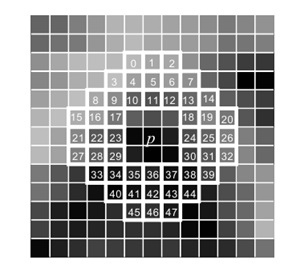According to the annealing simulation method, we define three functions:

• Cost function k. In our case, as a cost, we use repeatability. But there is one problem. Imagine that all points on each of the two images are detected as special. Then it turns out that the frequency is 100% absurd. On the other hand, let's have one singular point on two pictures, and these points coincided - the repeatability is also 100%, but this is not of interest to us either. And so the authors proposed to use as a quality criterion such:

\$\$ display \$\$ k = left {{{{{{{}}}} {right}}} {right} left ({1} sumlimits_ {i = 1} {{{left ({frac {{{d_i}}} {{{w_n}}}} right)} ^ 2}}} right) left ({1 + {{left {{frac {s} {{{w_s}}}} right)} ^ 2}} right) \$\$ display \$\$

r - repeatability,
\$ inline \$ {{d_i}} \$ inline \$
- number of detected angles on frame i, N - number of frames and s - tree size (number of vertices). W - configurable parameters of the method.]

• Function of temperature change with time:

\$\$ display \$\$ Tleft (I right) = beta exp left ({- frac {{alpha I}} {{{I_ {max}}}}} right) \$\$ display \$\$

where
\$ inline \$ alpha, beta \$ inline \$
- coefficients, Imax - number of iterations.

• A function that generates a new solution. The algorithm makes random tree modifications. First, select some vertex. If the selected vertex is a leaf of a tree, then with equal probability do the following:

We replace the vertex with a random subtree with depth 1

Change the class of this sheet (singular-non-singular point)

If this is NOT a sheet:

Replace the number of the test point with a random number from 0 to 47

Replace the vertex with a sheet with a random class

Swap two subtrees from this vertex

The probability P of making a change on iteration I is:

\$ inline \$ P = exp left (right) - kleft (i right)}} {T}} right) \$ inline \$

k - cost function, T - temperature, i - iteration number.

These modifications to the tree allow both the growth of the tree and its reduction. The method is random, it does not guarantee that the best tree will turn out. Start the method many times, choosing the best solution. In the original article, for example, run 100 times per 10?000 iterations each, which takes 200 hours of processor time. As the results show, it turns out in the end better than Tree FAST, especially on noisy pictures.

# Descriptor BRIEF

After the singular points are found, calculate their descriptors, i.e. Character sets characterizing the neighborhood of each singular point. BRIEF- A fast heuristic descriptor, is constructed from 256 binary comparisons between pixel brightnesses at blurred image. A binary test between the points x and y is defined as:

\$\$ display \$\$ tau left ({P, x, y} right): = left {{begin {array} {* {20} {c}} {1: pleft (x right) < pleft( y right)} {0:pleft( x right) ge pleft( y right)} end{array}} right.\$\$display\$\$In the original article, several ways of selecting points for binary comparisons were considered. As it turned out, one of the best ways is to select points randomly by the Gaussian distribution around the central pixel. This random sequence of points is chosen once and does not change in the future. The size of the considered neighborhood of the point is 31x31 pixels, and the blur aperture is equal to 5.

The resulting binary descriptor is resistant to light changes, perspective distortion, is quickly computed and compared, but is very unstable to rotation in the image plane.

# ORB - fast and effective

The development of all these ideas was the algorithm ORB (Oriented FAST and Rotated BRIEF), in which an attempt is made to improve the performance of BRIEF when the image is rotated. It was suggested first to calculate the orientation of a singular point and then to perform binary comparisons already in accordance with this orientationth. The algorithm works as follows:

1) Special points are detected using the fast tree FAST on the source image and on several images from the pyramid of thumbnail images.

2) For the detected points, the Harris measure is calculated, candidates with a low Harris measure value are discarded.

3) The angle of orientation of the singular point is calculated. To do this, first calculate the luminance moments for the neighborhood of the singular point:

\$ inline \$ {m_ {pq}} = sumlimits_ {x, y} {{x ^ p} {y ^ q} Ileft ({x, y} right)} \$ inline \$

x, y - pixel coordinates, I - brightness. And then the angle of orientation of the singular point:

\$ inline \$ theta = {rm {atan2}} left ({{m_ {01}}, {m_ {10}}} right) \$ inline \$

All these authors called the centroid orientation. As a result, we obtain a certain direction for the neighborhood of the singular point.

4) Having the orientation angle of the singular point, the sequence of points for the binary comparisons in the BRIEF descriptor is rotated in accordance with this angle, for example: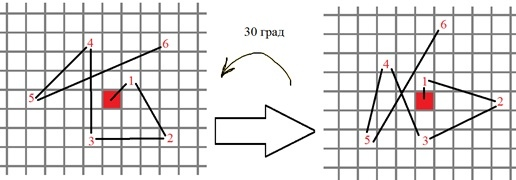More formally, the new positions for binary test points are calculated as:

\$\$ display \$\$ left ({begin {array} {* {20} {c}} {{x_i} '} {{y_i}'} end {array}} right) = Rleft (theta right) cdot left ( {begin {array} {* {20} {c}} {{x_i}} {{y_i}} end {array}} right) \$\$ display \$\$

5) The binary descriptor BRIEF
is calculated from the points obtained.

And on this not all! There is another interesting detail in the ORB, which requires separate explanations. The fact is that at the moment when we "turn" all the singular points to the zero angle, the random choice of binary comparisons in the descriptor ceases to be random. This leads to the fact that, firstly, some binary comparisons prove to be dependent among themselves; secondly, their average is no longer 0.5 (1 is lighter, 0 is darker, when the choice was random, then there was an average of 0.5) and all this significantly reduces the ability of the descriptor to distinguish between singular points.

The solution is to choose the "right" binary tests in the learning process, this idea has the same flavor as the tree training for the FAST-9 algorithm. Suppose we have a bunch of already found singular points. Let's consider all possible variants of binary tests. If the neighborhood is 31 x 3? and the binary test is a pair of subsets of 5 x 5 (due to blurring), then there are a lot of options for choosing N = (31-5) ^ 2. The algorithm for searching for "good" tests is as follows:

We calculate the result of all tests for all singular points

Order the entire set of tests for their distance from the average ???r3r3440.
Let's create a list that will contain the selected "good" tests, let's call the list R.

Add the first test from the sorted set
to R.
We take the next test and compare it with all tests in R. If the correlation is more than threshold, then discard the new test, otherwise we add it.

Repeat step 5 until we have typed in the required number of tests.

It turns out that the tests are selected so that, on the one hand, the average value of these tests was as close as possible to 0.? on the other hand, so that the correlation between the different tests was minimal. And this is what we need. Compare how it was and how it became: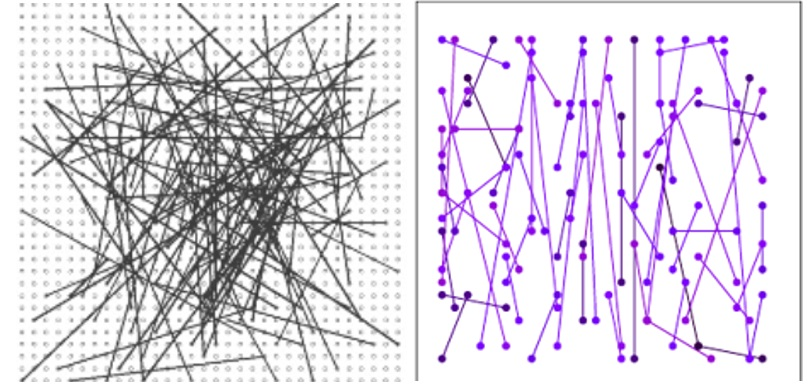Fortunately, the ORB algorithm is implemented in the OpenCV library in the cv :: ORB class. I'm using version ???. The class constructor takes the following parameters:

nfeatures is the maximum number of singular points

scaleFactor - multiplier for the image pyramid, more than one. The value 2 realizes the classical pyramid.

nlevels - the number of levels in the image pyramid.

edgeThreshold is the number of pixels at the border of the image where the singular points are not detected.

firstLevel - leave zero.

WTA_K is the number of points that is required for one descriptor element. If 2 is equal, then the brightness of two randomly selected pixels is compared. This is what you need.

scoreType - if ? then Harris is used as the measure of the feature, otherwise - the FAST measure (based on the sum of the differences in brightness units at the points of the circle). The FAST measure is slightly less stable, but works faster.

patchSize is the size of the neighborhood from which random pixels are selected for comparison. The code searches for and compares special points on two pictures, "templ.bmp" and "img.bmp"

Code [/b]
` cv :: Mat img_object = cv :: imread ("templ.bmp", 0);std :: vectorkeypoints_object, keypoints_scene;cv :: Mat descriptors_object, descriptors_scene;cv :: ORB orb (50? 1.? ? 3? ? ? ? 31);//singular points of the objectorb.detect (img_object, keypoints_object);orb.compute (img_object, keypoints_object, descriptors_object);//singular points of the picturecv :: Mat img = cv :: imread ("img.bmp", 1);cv :: Mat img_scene = cv :: Mat (img.size (), CV_8UC1);orb.detect (img, keypoints_scene);orb.compute (img, keypoints_scene, descriptors_scene);cv :: imshow ("desrs", descriptors_scene);cvWaitKey ();int test;for (int q = 0; q <10; q ++) test[q]= descriptors_scene.data[q];//- matching descriptor vectors using FLANN matchercv :: BFMatcher matcher;std :: vectormatches;cv :: Mat img_matches;if (! descriptors_object.empty () &&! descriptors_scene.empty ()) {matcher.match (descriptors_object, descriptors_scene, matches);double max_dist = 0; double min_dist = 100;//calculation of max and min idstance between keypointsfor (int i = 0; i < descriptors_object.rows; i++){double dist = matches[i].distance;if (dist < min_dist ) min_dist = dist;.if (dist> max_dist) max_dist = dist;}.//- Draw only good matchesstd :: vector < cv::DMatch > good_matches;for (int i = 0; i(), cv :: DrawMatchesFlags :: NOT_DRAW_SINGLE_POINTS);}.cv :: imshow ("match result", img_matches);cv :: waitKey ();.return 0; ` .
.

If someone helped to understand the essence of algorithms, then it's all for nothing. All the Habra.

References:

1. Fusing Points and Lines for High Performance Tracking

2. Machine learning for high-speed corner detection

3. Faster and better: a machine learning approach to corner detection

4. BRIEF: Binary Robust Independent Elementary Features

5. ORB: an ef fi cient alternative to SIFT or SURF
+ 0 -

#### Add comment

•••